Question

At 20C, the vapor pressure of pure benzene, C6H6 is 75 torr and that of pure toluene, C7H8, is 15 torr. Calculate the vapor pressure of a solution containing 39g benzene and 138g toluene. Both components in the solution are volatile.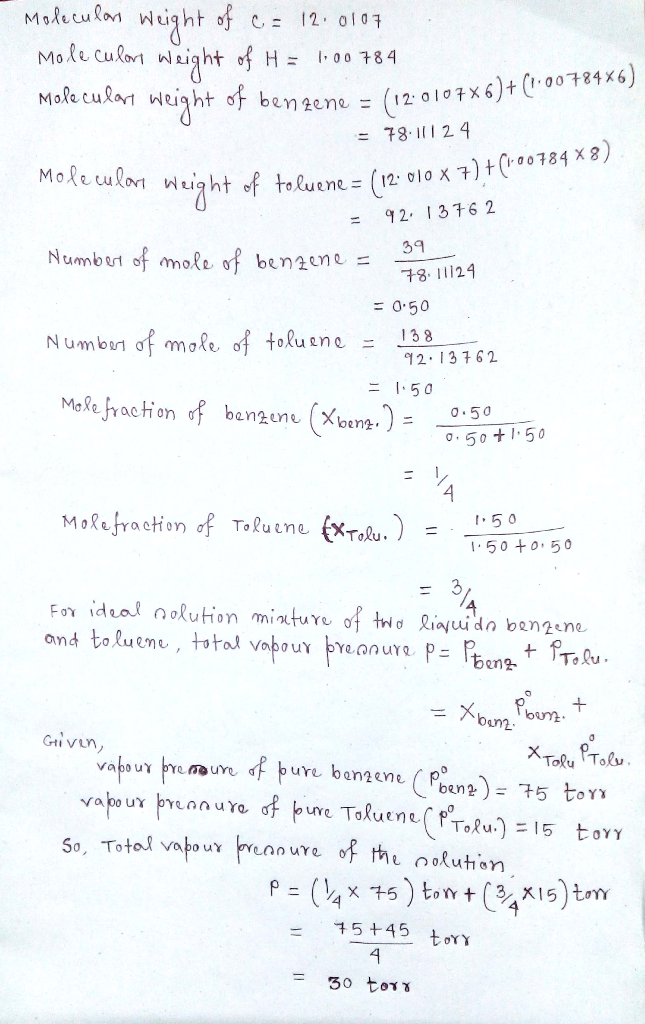#### Earn Coins

Coins can be redeemed for fabulous gifts.

Similar Homework Help Questions
• ### The vapor pressure of pure benzene (C6H6) at 25°C is 94.4 torrwhile the vapor pressure of...

The vapor pressure of pure benzene (C6H6) at 25°C is 94.4 torrwhile the vapor pressure of pure chloroform (CHCl3) at 25°C is 172 torr. An ideal solution isformed when 50 g of chloroform is added to 50 g of benzene. Calculate the vapor pressure of chloroform over thischloroform-benzene solution at 25°C. a) 86 torr b) 68 torr c) 148 torr d) 125 torr e) 172 torr

• ### A solution of benzene (C6H6) and toluene (C7H8) is 29.0 % benzene by mass. At 25∘C...

A solution of benzene (C6H6) and toluene (C7H8) is 29.0 % benzene by mass. At 25∘C the vapor pressures of pure benzene and pure toluene are 94.2 and 28.4 torr, respectively. Part A Assuming ideal behavior, calculate the vapor pressure of benzene in the mixture. Express the pressure to three significant figures and include the appropriate units. Part B Assuming ideal behavior, calculate the vapor pressure of toluene in the mixture. Express the pressure to three significant figures and include...

• ### Vapor Pressure

What are the partial and total vapor pressure of a solution obtained by mixing 35.8 g benzene, C6H6, and 56.7 g toluene,C7H8, at 25 C? What is the mole fraction ofbenzene in the liquid phase? What is the mole fraction of benzene in the vapor phase? Vapor pressure at 25C are 95.1 torr for benzene and 28.4 torr for toluene.

• ### If at a given temperature, the vapor pressure above pure benzene is 200.0 Torr and above...

If at a given temperature, the vapor pressure above pure benzene is 200.0 Torr and above pure toluene is 40.50 Torr and the mole fraction os toluene is 0.575, what is the partial pressure of each gas above the solution and what is the mole fraction of each gas in the vapor?

• ### 1) Benzene and toluene form nearly ideal solutions. At 20°C the vapor pressure of pure benzene...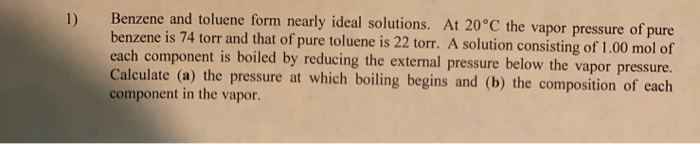1) Benzene and toluene form nearly ideal solutions. At 20°C the vapor pressure of pure benzene is 74 torr and that of pure toluene is 22 torr. A solution consisting of 1.00 mol of each component is boiled by reducing the external pressure below the vapor pressure. Calculate (a) the pressure at which boiling begins and (b) the composition of each component in the vapor.

• ### Separate and Condense Condensed vapor YB1 = 0.714 The vapor pressure of pure benzene is 750.0...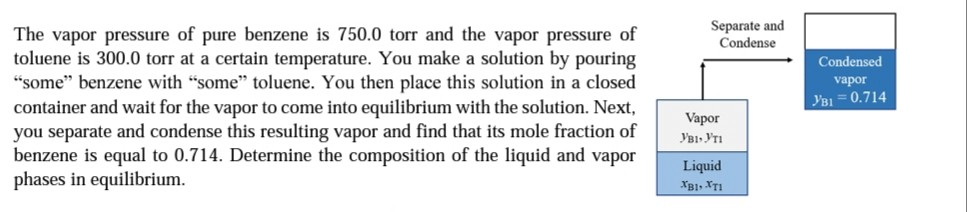Separate and Condense Condensed vapor YB1 = 0.714 The vapor pressure of pure benzene is 750.0 torr and the vapor pressure of toluene is 300.0 torr at a certain temperature. You make a solution by pouring "some" benzene with "some" toluene. You then place this solution in a closed container and wait for the vapor to come into equilibrium with the solution. Next, you separate and condense this resulting vapor and find that its mole fraction of benzene is equal...

• ### At 25C, the vapor pressure of pure benzene is 0.1252 atm. When 6.50 g of a...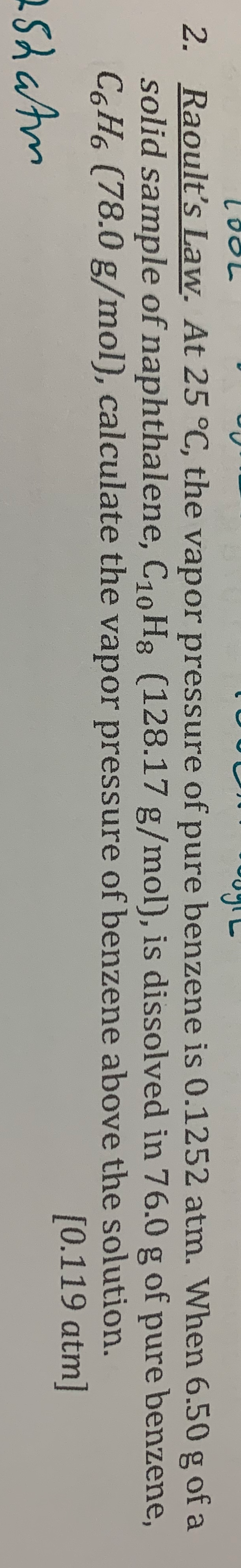At 25C, the vapor pressure of pure benzene is 0.1252 atm. When 6.50 g of a sold sample of naphthalene,C10H8(128.17 g/mol), is dissolved in 76.0 g of pure benzene, C6H6, calculate the vapor pressure of benzene above the solution oy looL 2. Raoult's Law. At 25 °C, the vapor pressure of pure benzene is 0.1252 atm. When 6.50 g of a solid sample of naphthalene, C10Hg (128.17 g/mol), is dissolved in 76.0 g of pure benzene, C6H6 (78.0 g/mol), calculate...

• ### Benzene, C6H6, and octane, C8H14, form an ideal solution. At 60°C the vapor pressure of pure...

Benzene, C6H6, and octane, C8H14, form an ideal solution. At 60°C the vapor pressure of pure benzene is 0.507 atm, and the vapor pressure of pure octane is 0.103 atm. A solution is composed of 3.53 g of benzene, and 40.2 g of octane. What is the mole fraction of benzene in the vapor phase above the liquid? In order to receive full credit, your work should clearly show the following: a) the calculation of the partial pressure of benzene...

• ### 2. Raoult's Law. At 25 °C, the vapor pressure of pure benzene is 0.1252 atm. When...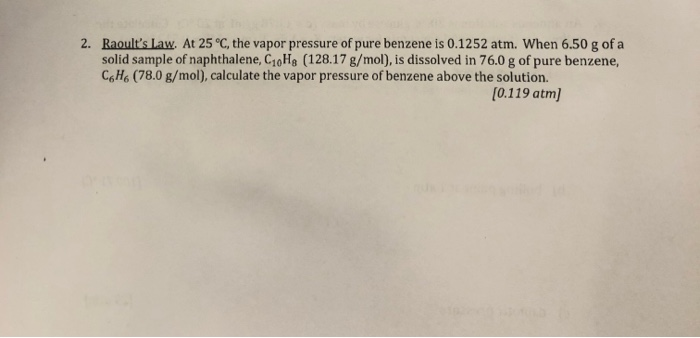2. Raoult's Law. At 25 °C, the vapor pressure of pure benzene is 0.1252 atm. When 6.50 g of a solid sample of naphthalene, C10Hg (128.17 g/mol), is dissolved in 76.0 g of pure benzene, C6H6 (78.0 g/mol), calculate the vapor pressure of benzene above the solution. [0.119 atm]

• ### The vapor pressure of pure water at 25.0 °C is 23.76 torr. The vapor pressure of...

The vapor pressure of pure water at 25.0 °C is 23.76 torr. The vapor pressure of a solution containing 5.40 g of a nonvolatile substance in 90.0 g of water is 23.32 torr. What is the molecular weight of the solute?